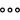# Integrating Custom Calculations and Forecast Layout

You can add custom calculations to the Forecast or Rolling Forecast layout, which allows you to combine different values in the same table. You can also create Calculation Input Controls for your forecast.

Using the Calculation Editor, you can create a Forecast member that is added to your table. When you add more than one calculated Forecast or Rolling Forecast member, you can then create a custom calculation to show the delta between those members.

Use an Input Control for a Forecast

You can add input controls to your tables for forecast layouts.

To create an Input Control for a forecast, do the following:

1. Under Rows or Columns in the Builder tab, select Add Measures/Dimensions and add the Cross Calculations dimension.

2. In the Cross Calculations dimension, selectAdd Calculation.

3. From the Calculation Editor Type, select Forecast.

4. From the Properties section, under Version, select Create a New Calculation Input Control.

5. Provide a name for the input control.

6. In the Input Values section, select Click to Add Values.

7. Select your forecast versions and then click OK; click OK again.

The Calculation Input Control is created.

8. To close the Calculation Editor, click OK.